# Additive mappings on ${C}^{\ast }$-algebras sub-preserving absolute values of products

## Abstract

Let $\mathcal{A}$ be a ${C}^{\ast }$-algebra of real rank zero and $\mathcal{B}$ be a ${C}^{\ast }$-algebra with unit I. It is shown that if $\varphi :\mathcal{A}⟶\mathcal{B}$ is an additive mapping which satisfies $|\varphi \left(A\right)\varphi \left(B\right)|\le \varphi \left(|AB|\right)$ for every $A,B\in {\mathcal{A}}_{+}$ and $\varphi \left(A\right)=I$ for some $A\in {\mathcal{A}}_{s}$ with $\parallel A\parallel \le 1$, then the restriction of mapping ϕ to ${\mathcal{A}}_{s}$ is a Jordan homomorphism, where ${\mathcal{A}}_{s}$ denotes the set of all self-adjoint elements. We will also show that if ϕ is surjective preserving the product and an absolute value, then ϕ is a $\mathbb{C}$-linear or $\mathbb{C}$-antilinear -homomorphism on $\mathcal{A}$.

MSC:47B49, 46L05, 47L30.

## 1 Introduction and preliminaries

In recent years, the subject of linear preserver problems is the focus of attention of many mathematicians, and much research has been going on in this area. Here we refer to the articles [16, 916].

In what follows, let $\mathcal{A}$ and $\mathcal{B}$ be two ${C}^{\ast }$-algebras with unit I. We say that a mapping $\varphi :\mathcal{A}⟶\mathcal{B}$ is preserving (resp. sub-preserving) absolute values of a product if $|\varphi \left(A\right)\varphi \left(B\right)|=\varphi \left(|AB|\right)$ (resp. $|\varphi \left(A\right)\varphi \left(B\right)|\le \varphi \left(|AB|\right)$) for every $A,B\in \mathcal{A}$, where ${|A|}^{2}={A}^{\ast }A$. By a -homomorphism we just mean a map $\varphi :\mathcal{A}⟶\mathcal{B}$ which preserves the ring structure and for which $\varphi \left({A}^{\ast }\right)=\varphi {\left(A\right)}^{\ast }$ for every $A\in \mathcal{A}$. A map $\varphi :\mathcal{A}\to \mathcal{B}$ is said to be a Jordan -homomorphism if it is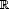-linear, $\varphi \left({A}^{\ast }\right)=\varphi {\left(A\right)}^{\ast }$ and $\varphi {\left(A\right)}^{2}=\varphi \left({A}^{2}\right)$ for all $A\in \mathcal{A}$. We also say a map $\varphi :\mathcal{A}\to \mathcal{B}$ is unital if $\varphi \left(I\right)=I$. The class of all self-adjoint elements in a ${C}^{\ast }$-algebra $\mathcal{A}$ is denoted by ${\mathcal{A}}_{s}$. We define $A\circ B=\frac{1}{2}\left[AB+BA\right]$ for all $A,B\in {\mathcal{A}}_{s}$. It is known that $\left({\mathcal{A}}_{s},+,\circ \right)$ is a ${C}^{\ast }$-algebra which is called a Jordan algebra.

In , Molnar considered bijective mappings ϕ from a von Neumann algebra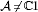which is a factor onto a von Neumann algebra which preserves a product and an absolute value. He showed ϕ is of the form

$\varphi \left(A\right)=\tau \left(A\right)\psi \left(A\right)\phantom{\rule{1em}{0ex}}\left(A\in \mathcal{A}\right),$

where ψ is either a linear or conjugate linear *-algebra isomorphism and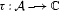is a scalar function of Modulus 1.

It is the aim of this paper to continue this work by studying additive mappings ϕ from a ${C}^{\ast }$-algebra of real rank zero into a ${C}^{\ast }$-algebra that sub-preserve product and absolute value. In fact, we show that if the mapping ϕ which is an additive sub-preserving product absolute value map from a ${C}^{\ast }$-algebra $\mathcal{A}$ into a ${C}^{\ast }$-algebra then ϕ is a contraction. Moreover, if $\mathcal{A}$ is a ${C}^{\ast }$-algebra of real rank zero and $\varphi \left(A\right)=I$ for some A in the closed unit ball ${\left({\mathcal{A}}_{s}\right)}_{1}$, then the restriction of mapping ϕ to ${\mathcal{A}}_{s}$ is a Jordan homomorphism. We will also show that if ϕ is a surjective preserving product and absolute value and $\mathcal{A}$ is a ${C}^{\ast }$-algebra of real rank zero, then ϕ is a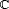-linear or-antilinear -homomorphism on $\mathcal{A}$. All we need about ${C}^{\ast }$-algebras and von Neumann algebras can be found in [7, 8].

## 2 The main results

Firstly, we need some auxiliary lemmas to prove our main result.

Lemma 2.1 Let$\mathcal{A}$and$\mathcal{B}$be two unital${C}^{\ast }$-algebras with unit I. If$\varphi :\mathcal{A}⟶\mathcal{B}$is a map satisfying

$|\varphi \left(A\right)\varphi \left(B\right)|\le \varphi \left(|AB|\right)\phantom{\rule{1em}{0ex}}\left(\mathrm{\forall }A,B\in {\mathcal{A}}_{+}\right)$
(2.1)

then ϕ preserves positive elements. Moreover, if$\varphi \left(0\right)=0$, then for all$A,B\in {\mathcal{A}}_{+}$we have

$AB=BA=0\phantom{\rule{1em}{0ex}}⇒\phantom{\rule{1em}{0ex}}\varphi \left(A\right)\varphi \left(B\right)=\varphi \left(B\right)\varphi \left(A\right)=0.$
(2.2)

Proof If A is a positive element in $\mathcal{A}$, then $|\varphi \left(A\right)\varphi \left(I\right)|\le \varphi \left(A\right)$. This means $\varphi \left(A\right)$ is positive and preserves positive elements.

Let $A,B\in {\mathcal{A}}_{+}$ and $AB=BA=0$. By the assumption $|\varphi \left(A\right)\varphi \left(B\right)|\le \varphi \left(|AB|\right)=0$. Thus $|\varphi \left(A\right)\varphi \left(B\right)|=0$ and hence $\varphi \left(A\right)\varphi \left(B\right)=\varphi \left(B\right)\varphi \left(A\right)=0$. □

Lemma 2.2 Let$\mathcal{A}$and$\mathcal{B}$be two unital${C}^{\ast }$-algebras with unit I. If$\varphi :\mathcal{A}⟶\mathcal{B}$is an additive mapping satisfying (2.1), then ϕ is order preserving and contraction (i.e. $\parallel \varphi \left(A\right)\parallel \le \parallel A\parallel$) on$\left({\mathcal{A}}_{s},+,\circ \right)$.

Proof By Lemma 2.1 ϕ preserves positive elements. Hence additivity of ϕ implies that ϕ is order preserving. And also, since every self-adjoint element is the difference of two positive elements, ϕ preserves self-adjoint elements. Indeed, we show that ϕ maps the part of positive (resp. negative) of A to the part of positive (resp. negative) of $\varphi \left(A\right)$. In fact, $\varphi \left({A}_{+}\right)=\varphi {\left(A\right)}_{+}$ and $\varphi \left({A}_{-}\right)=\varphi {\left(A\right)}_{-}$, where, $A={A}_{+}-{A}_{-}$ and ${A}_{+}{A}_{-}=0={A}_{-}{A}_{+}$. We just need to show $\varphi \left({A}_{+}\right)\varphi \left({A}_{-}\right)=0=\varphi \left({A}_{-}\right)\varphi \left({A}_{+}\right)$ because the decomposition of $\varphi \left(A\right)$ is unique and ϕ preserves positives. Applying Lemma 2.1 and the equation ${A}_{+}{A}_{-}=0={A}_{-}{A}_{+}$, we get the assertion.

The proof of-linearity of ϕ is similar to the first step of the proof of , Theorem 1]. The details are omitted.

Now, we show ϕ is contraction on $\left({\mathcal{A}}_{s},+,\circ \right)$. If A is a self-adjoint element. We can write $A={A}_{+}-{A}_{-}$, where ${A}_{+}$ and ${A}_{-}$ are positive elements. We have

$\begin{array}{rcl}\varphi {\left(A\right)}^{2}& =& \varphi {\left({A}_{+}-{A}_{-}\right)}^{2}=\varphi {\left({A}_{+}\right)}^{2}+\varphi {\left({A}_{-}\right)}^{2}\\ \le & \varphi \left({{A}_{+}}^{2}\right)+\varphi \left({{A}_{-}}^{2}\right)=\varphi \left({{A}_{+}}^{2}+{{A}_{-}}^{2}\right)\\ =& \varphi \left({\left({A}_{+}-{A}_{-}\right)}^{2}\right)=\varphi \left({A}^{2}\right).\end{array}$

Since ϕ is order preserving, observe

$\varphi {\left(A\right)}^{2}\le \varphi \left({A}^{2}\right)\le \parallel {A}^{2}\parallel \varphi \left(I\right),$

because ${A}^{2}\le \parallel {A}^{2}\parallel I$. It implies that

${\parallel \varphi \left(A\right)\parallel }^{2}=\parallel \varphi {\left(A\right)}^{2}\parallel \le {\parallel A\parallel }^{2},$

since $\parallel \varphi \left(I\right)\parallel \le 1$. Taking square root, we obtain $\parallel \varphi \left(A\right)\parallel \le \parallel A\parallel$, which yields ϕ is a contraction on $\left({\mathcal{A}}_{s},+,\circ \right)$. □

Lemma 2.3 Let$\mathcal{A}$and$\mathcal{B}$be two unital${C}^{\ast }$-algebras with unit I. If$\varphi :\mathcal{A}⟶\mathcal{B}$is an additive map satisfying (2.1) and

$\varphi \left(U\right)=I,\phantom{\rule{1em}{0ex}}\mathit{\text{for some}}U\in {\left({\mathcal{A}}_{s}\right)}_{1},$
(2.3)

then ϕ is unital.

Proof By the hypothesis, there exists an operator $U\in {\left({\mathcal{A}}_{s}\right)}_{1}$ such that $\varphi \left(U\right)=I$. Since ϕ is order preserving by Lemma 2.2 and $|A|\le \parallel A\parallel I$ for every $A\in \mathcal{A}$, we have

$I=\varphi {\left(U\right)}^{2}\le \varphi \left({U}^{2}\right)\le {\parallel U\parallel }^{2}\varphi \left(I\right)\le \varphi \left(I\right).$

On the other hand, we have

$\varphi \left(I\right)\le \parallel \varphi \left(I\right)\parallel I\le I,$

because ϕ is contraction by Lemma 2.2. Therefore, $\varphi \left(I\right)=I$. □

The following example shows that the condition (2.3) in Lemma 2.3 is necessary.

Example 2.4 Define an additive mapping $\varphi :C\left[0,1\right]⟶C\left[0,1\right]$ by

$\varphi \left(f\right)=r\cdot f$

for all $f\in C\left[0,1\right]$, where $r\left(t\right)=\frac{1+t}{2}$. Note that r is positive and ${r}^{2}\le r$. Obviously, ϕ is an additive mapping satisfying

$|\varphi \left(f\right)\varphi \left(g\right)|={r}^{2}|fg|\le r|fg|=\varphi \left(|fg|\right),$

for every $f,g\in C\left[0,1\right]$, but clearly there is not any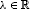such that $\varphi \left(I\right)=\lambda I$ because $\varphi \left(I\right)=r$.

Lemma 2.5 Let$\mathcal{A}$be a${C}^{\ast }$-algebra of real rank zero and$\mathcal{B}$be a unital${C}^{\ast }$-algebra with unit I. If$\varphi :\mathcal{A}⟶\mathcal{B}$is an additive mapping satisfying (2.1), then

1. (i)

for every self-adjoint operator $A\in \mathcal{A}$, we have

$\varphi {\left(A\right)}^{2}=\varphi \left({A}^{2}\right)\varphi \left(I\right)=\varphi \left(I\right)\varphi \left({A}^{2}\right).$
(2.4)
2. (ii)

$N=\left\{A\in {\mathcal{A}}_{s}:\varphi \left(A\right)=0\right\}$ is a closed ideal of $\left({\mathcal{A}}_{s},+,\circ \right)$.

Proof (i) Let E and F be mutually orthogonal projections. By Lemma 2.1 $\varphi \left(E\right)\varphi \left(F\right)=\varphi \left(F\right)\varphi \left(E\right)=0$, in particular, $\varphi \left(I-E\right)\varphi \left(E\right)=\varphi \left(E\right)\varphi \left(I-E\right)=0$. That is, $\varphi {\left(E\right)}^{2}=\varphi \left(E\right)\varphi \left(I\right)=\varphi \left(I\right)\varphi \left(E\right)$.

Assume that $A\in \mathcal{A}$ is of the form ${\sum }_{j=1}^{n}{\lambda }_{j}{E}_{j}$ for some scalerand finitely many mutually orthogonal projections ${E}_{j}$, then

$\begin{array}{rcl}\varphi {\left(A\right)}^{2}& =& {\left[\varphi \left(\sum _{j=1}^{n}{\lambda }_{j}{E}_{j}\right)\right]}^{2}=\sum _{j=1}^{n}{\lambda }_{j}^{2}\varphi {\left({E}_{j}\right)}^{2}\\ =& \sum _{j=1}^{n}{\lambda }_{j}^{2}\varphi \left({E}_{j}\right)\varphi \left(I\right)=\sum _{j=1}^{n}{\lambda }_{j}^{2}\varphi \left(I\right)\varphi \left({E}_{j}\right)\\ =& \varphi \left({A}^{2}\right)\varphi \left(I\right)=\varphi \left(I\right)\varphi \left({A}^{2}\right).\end{array}$

Now, assume A is an arbitrary self-adjoint element. Since $\mathcal{A}$ is a ${C}^{\ast }$-algebra with real rank zero, its every self-adjoint element can be approximated by the elements of the above form. Hence the continuity of ϕ entails

$\varphi {\left(A\right)}^{2}=\varphi \left({A}^{2}\right)\varphi \left(I\right)=\varphi \left(I\right)\varphi \left({A}^{2}\right),$

for every self-adjoint operator $A\in \mathcal{A}$.

1. (ii)

Let $A\in \mathcal{A}$ be a self-adjoint element such that $\varphi \left(I\right)\varphi \left(A\right)=0=\varphi \left(A\right)\varphi \left(I\right)$. We show that $\varphi \left(A\right)=0$.

Multiplying through equation (2.4) by $\varphi \left(I\right)$ (on the left) we get $0=\varphi \left(I\right)\varphi {\left(A\right)}^{2}=\varphi {\left(I\right)}^{2}\varphi \left({A}^{2}\right)$. Since $\varphi \left(I\right)$ and $\varphi \left({A}^{2}\right)$ commute and by the assumption, we have $0=\varphi \left(I\right)\varphi {\left(A\right)}^{2}=\varphi {\left(I\right)}^{2}\varphi \left({A}^{2}\right)\ge \varphi {\left(I\right)}^{2}\varphi {\left(|A|\right)}^{2}$. This implies that

$0=\varphi \left(I\right)\varphi \left(|A|\right).$
(2.5)

Since ${A}^{2}\le \parallel A\parallel |A|$, by using the order preserving property of ϕ we yield $\varphi {\left(A\right)}^{2}=\varphi \left(I\right)\varphi \left({A}^{2}\right)=\varphi \left(I\right)\varphi \left({|A|}^{2}\right)\le \varphi \left(I\right)\varphi \left(\parallel A\parallel |A|\right)=\parallel A\parallel \varphi \left(I\right)\varphi \left(|A|\right)=0$. So $\varphi \left(A\right)=0$ because $\varphi \left(A\right)$ is a self-adjoint element.

It follows N is a closed ideal of $\left({\mathcal{A}}_{s},+,\circ \right)$ by the step 4 of , Theorem 2.1]. □

In the following theorem we would like to characterize the Jordan homomorphisms ϕ which are additive mappings sub-preserving a product and an absolute value.

Theorem 2.6 Let$\mathcal{A}$be a${C}^{\ast }$-algebra of real rank zero and$\mathcal{B}$be a unital${C}^{\ast }$-algebra with unit I. If$\varphi :\mathcal{A}⟶\mathcal{B}$is an additive mapping satisfying (2.1) and (2.3), then the restriction of the map ϕ to${\mathcal{A}}_{s}$is a Jordan homomorphism.

Proof According to Lemma 2.3 and Lemma 2.5(i) we yield the statement. □

Now we also show that the condition (2.3) in Theorem 2.6 is necessary.

Example 2.7 The same as in Example 2.4, let $\mathcal{A}$ be a von Neumann algebra with a non trivial center. Define an additive mapping $\varphi :\mathcal{A}⟶\mathcal{A}$ by

$\varphi \left(A\right)=UA,$

where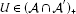is invertible, ${U}^{2} and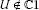. Obviously, ϕ is an additive mapping that satisfies in (2.1), but no nonzero multiple of ϕ is a Jordan homomorphism, because if $\psi \left(A\right)=\lambda \varphi \left(A\right)$ with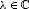is a Jordan homomorphism, then we obtain ${U}^{2}={\lambda }^{2}$, that is a contradiction.

In the following theorem we show that if $\varphi \left(I\right)$ is an injective operator and ϕ is an additive map which satisfies in (2.1), then the restriction of ϕ is a Jordan homomorphism multiplied by $\varphi \left(I\right)$.

Theorem 2.8 Let$\mathcal{A}$be a${C}^{\ast }$-algebra of real rank zero and$\mathcal{B}$be a unital${C}^{\ast }$-algebra with unit I. If$\varphi :\mathcal{A}⟶\mathcal{B}$is an additive mapping satisfying$|\varphi \left(A\right)\varphi \left(B\right)|\le \varphi \left(|AB|\right)$for every$A,B\in {\mathcal{A}}_{+}$and$\varphi \left(I\right)$is an injective operator, then the restriction of mapping$\psi :\mathcal{A}⟶\mathcal{B}$is defined by$\varphi \left(A\right)=\varphi \left(I\right)\psi \left(A\right)$, to${\mathcal{A}}_{s}$is a Jordan homomorphism.

Proof Injectivity of $\varphi \left(I\right)$ implies ψ is well defined. Let $A\in {\mathcal{A}}_{s}$. By applying Lemma 2.5, we can show $\varphi \left(A\right)\varphi \left(I\right)=\varphi \left(I\right)\varphi \left(A\right)$. By the definition of ψ, we have

$\begin{array}{r}\varphi {\left(I\right)}^{2}\psi \left(A\right)=\varphi \left(I\right)\psi \left(A\right)\varphi \left(I\right),\\ \varphi \left(I\right)\left(\varphi \left(I\right)\psi \left(A\right)-\psi \left(A\right)\varphi \left(I\right)\right)=0,\\ \varphi \left(I\right)\psi \left(A\right)=\psi \left(A\right)\varphi \left(I\right).\end{array}$

This means $\varphi \left(I\right)$ commutes with $\psi \left(A\right)$ for every self-adjoint operator $A\in \mathcal{A}$. Again, by using Lemma 2.5, we yield

$\begin{array}{r}\varphi {\left(I\right)}^{2}\psi \left({A}^{2}\right)=\varphi \left(I\right)\varphi \left({A}^{2}\right)\\ \phantom{\varphi {\left(I\right)}^{2}\psi \left({A}^{2}\right)}=\varphi {\left(A\right)}^{2}=\varphi {\left(I\right)}^{2}\psi {\left(A\right)}^{2},\\ \varphi {\left(I\right)}^{2}\left(\psi \left({A}^{2}\right)-\psi {\left(A\right)}^{2}\right)=0,\\ \psi \left({A}^{2}\right)=\psi {\left(A\right)}^{2}.\end{array}$

This completes the proof. □

In , Molnar considered bijective mappings ϕ from a von Neumann algebrawhich is a factor onto a von Neumann algebra which preserves a product and an absolute value. He showed ϕ is of the form

$\varphi \left(A\right)=\tau \left(A\right)\psi \left(A\right)\phantom{\rule{1em}{0ex}}\left(A\in \mathcal{A}\right),$

where, ψ is either a linear or conjugate linear -algebra isomorphism andis a scalar function of Modulus 1. Below, we present the result where we do not assume injectivity but ϕ is an additive map from ${C}^{\ast }$-algebra $\mathcal{A}$ onto a ${C}^{\ast }$-algebra of real rank zero which preserves a product and an absolute value, and it is shown ϕ is a-linear or-antilinear -homomorphism.

Theorem 2.9 Let$\mathcal{A}$and$\mathcal{B}$be two unital${C}^{\ast }$-algebra with unit I. If$\varphi :\mathcal{A}⟶\mathcal{B}$is an additive mapping satisfying$|\varphi \left(A\right)\varphi \left(B\right)|=\varphi \left(|AB|\right)$for every$A,B\in \mathcal{A}$and$\varphi \left(A\right)=I$for some$A\in \mathcal{A}$, then ϕ is unital and the restriction of mapping ϕ to${\mathcal{A}}_{S}$is a Jordan homomorphism. Moreover, if ϕ is surjective and$\mathcal{B}$be a${C}^{\ast }$-algebra of real rank zero then, ϕ is a-linear or-antilinear -homomorphism.

Proof By Lemma 2.2 ϕ preserves self-adjoint element. So $\varphi {\left(A\right)}^{2}=\varphi \left({A}^{2}\right)$, for all of self-adjoint elements A, in particular, ϕ preserves projection. By the hypothesis, there is an element $U\in \mathcal{A}$ such that $\varphi \left(U\right)=I$. Then,

$I=\varphi \left(U\right)=|\varphi \left(U\right)\varphi \left(U\right)|=\varphi \left(|{U}^{2}|\right).$

Thus without loss of generality we can assume U is a positive element. Now, we have

$I=\varphi \left(U\right)=\varphi \left(|UI|\right)=|\varphi \left(U\right)\varphi \left(I\right)|=|\varphi \left(I\right)|=\varphi \left(I\right).$

So ϕ is a unital map.

And also we have

$|\varphi \left(A\right)|=|\varphi \left(A\right)\varphi \left(I\right)|=\varphi \left(|A|\right)\phantom{\rule{1em}{0ex}}\left(A\in \mathcal{A}\right).$

Therefore, ϕ is a-linear or-antilinear -homomorphism on $\mathcal{A}$ by , Theorem 2.5]. □

## References

1. Aupetit B, du Toit Mouton H: Spectrum preserving linear mappings in Banach algebras. Stud. Math. 1994, 109: 91–100.

2. Brešar M: Commuting traces of biadditive mappings, commutativity-preserving, mappings and Lie mappings. Trans. Am. Math. Soc. 1993, 335: 525–546.

3. Chan GH, Lim MH: Linear preservers on powers of matrices. Linear Algebra Appl. 1992, 162–164: 615–626.

4. Choi MD, Jafarian AA, Radjavi H: Linear maps preserving commutativity. Linear Algebra Appl. 1987, 87: 227–241.

5. Jafarian AA, Sourour AR: Spectrum-preserving linear maps. J. Funct. Anal. 1986, 66: 255–261. 10.1016/0022-1236(86)90073-X

6. Gudder S, Nagy G: Quentially independent effects. Proc. Am. Math. Soc. 2002, 130: 1125–1130. 10.1090/S0002-9939-01-06194-9

7. Kadison RV, Ringrose JR: Fundamentals of the Theory of Operator Algebras: Elementary Theory. Academic Press, New York; 1997.

8. Kadison RV, Ringrose JR: Fundamentals of the Theory of Operator Algebras: Advanced Theory. Academic Press, Orlando; 1997.

9. Li CK, Tsing NK: Linear preserver problems: a brief introduction and some special techniques. Linear Algebra Appl. 1992, 162–164: 217–235.

10. Molnár L:Two characterisations of additive *-automorphisms of $B\left(H\right)$. Bull. Aust. Math. Soc. 1996, 53: 391–400. 10.1017/S0004972700017147

11. Molnár L: Multiplicative Jordan triple isomorphisms on the selfadjoint elements of von Neuman algebras. Linear Algebra Appl. 2006, 419: 586–600. 10.1016/j.laa.2006.06.007

12. Omladič M: On operators preserving commutativity. J. Funct. Anal. 1986, 66: 105–122. 10.1016/0022-1236(86)90084-4

13. Omladič M, Šemrl P: Linear mappings that preserve potent operators. Proc. Am. Math. Soc. 1995, 123: 1069–1074.

14. Radjabalipour M: Additive mappings on von Neumann algebras preserving absolute values. Linear Algebra Appl. 2003, 368: 229–241.

15. Radjabalipour M, Seddighi K, Taghavi Y: Additive mappings on operator algebras preserving absolute values. Linear Algebra Appl. 2001, 327: 197–206. 10.1016/S0024-3795(00)00338-4

16. Śemrl P: Linear mappings preserving square-zero matrices. Bull. Aust. Math. Soc. 1993, 48: 365–370. 10.1017/S0004972700015811

17. Taghavi A:Additive mappings on ${C}^{\ast }$-algebras preserving absolute values. Linear Multilinear Algebra 2012, 60(1):33–38. 10.1080/03081087.2010.533271

## Acknowledgement

This research is partially supported by the Research Center in Algebraic Hyperstructures and Fuzzy Mathematics, University of Mazandaran, Babolsar, Iran.

## Author information

Authors

### Corresponding author

Correspondence to Ali Taghavi.

### Competing interests

The author declares that they have no competing interests.

## Rights and permissions

Reprints and Permissions

Taghavi, A. Additive mappings on ${C}^{\ast }$-algebras sub-preserving absolute values of products. J Inequal Appl 2012, 161 (2012). https://doi.org/10.1186/1029-242X-2012-161

• Accepted:

• Published:

• DOI: https://doi.org/10.1186/1029-242X-2012-161

### Keywords

• ${C}^{\ast }$-algebra
• $\mathbb{C}$-linear
• $\mathbb{C}$-antilinear
• homomorphism
• linear preserver problem
• real rank zero